Courses

# Example Problem On Humidification Chemical Engineering Notes | EduRev

## Chemical Engineering : Example Problem On Humidification Chemical Engineering Notes | EduRev

The document Example Problem On Humidification Chemical Engineering Notes | EduRev is a part of the Chemical Engineering Course Mass Transfer.
All you need of Chemical Engineering at this link: Chemical Engineering

HUMIDIFICATION AND AIR CONDITIONING
Example Problem 6.2:
It is planned to cool water from 43.30C to 29.40C in a packed countercurrent water-cooling tower using entering air at 29.40C with a wet bulb temperature of 23.90C. The water flow is 9764.9 kg/h.m2 and the air flow is 6835.4 kg/h.m2 . The overall mass transfer coefficient is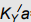=2500 kg/m3h (ΔY/ ). Calculate (a) minimum air rate that can be used and (b) tower height needed if air flow of 6835.4 kg/h.m2 is used.
Given: Height of transfer unit, HtoG=Gs/a. Tie lines are vertical.
Enthalpies of saturated air-water vapor (Base temperature: 00C)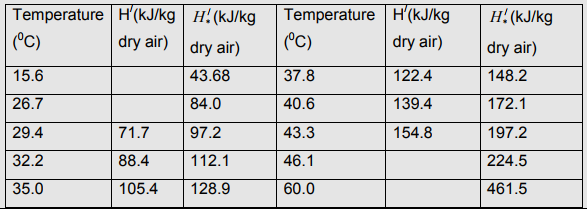Solution 6.2:

Y/1 =0.0165 kg/kg dry air [From Psychrometric chart]
H/1 =71.7 kJ/kg
H/2 =154.8 kJ/kg
From Graph, at Gs,min, H/2 =197 kJ/kg at 43.30
(a) Gs, min=4546.4 kg/h.m2 .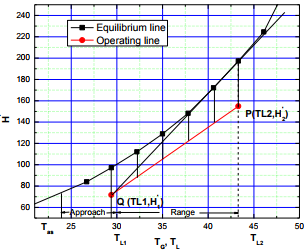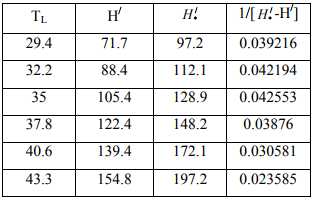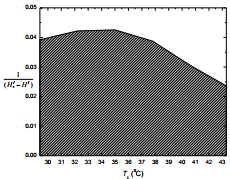NtoG=Area under the curve= (154.8-71.7)×0.036148=3.004
HtoG=2.734 m

(b)Tower height= 2.734×3.004 m=8.213 m (Ans.)

Example Problem 6.3:
A cooling tower of 50 m2 cross-sectional area is required to cool the warm water from 420C to 290C at a rate of 425250 kg/h. The ambient air at 320C has a wet-bulb temperature of 220C and air rate (moist) is 6000 kg/h.m2 . The overall mass transfer coefficient,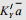=740.375 kg/m3h (ΔY/ ) where a is specific interfacial area of air-water contact. Determine (a) the minimum air rate and (b) overall gas-phase enthalpy transfer units. (c) Keeping other conditions unchanged, if the wet-bulb temperature is changed to 25.50C, what will the cold water temperature?
Given: Antoine Equation: ln PV(bar) =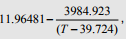temperature in K. Total pressure is 1 atm.

Solution 6.3: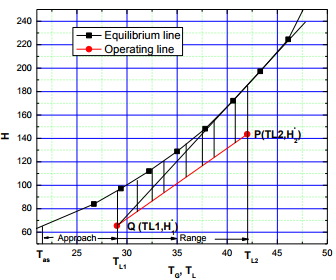TG1=32ºC, Tw1=22ºC, From psychrometric chart, Y/1 = 0.013 kg/ kg dry air
H/1 = 65.44 kJ / kg
(a) Draw tangent to equilibrium curve from point Q.
Slope of the operating line for minimum air rate: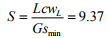L=425250/50 kg/h.m2 =8505 kg/h.m2
cwL=4.187 kJ/kgºC
Gsmin=3800.47 kg/h.m2
Gs=6000/(1+0.013) kg/h.m2 =5923 kg/h.m2
Slope of the operating line for actual air rate: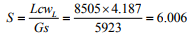Draw operating line with slope 6.006 through point Q. TL2=42ºC. Locate point P. (Get H/2 from graph).
or,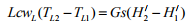8505 X 4.187(42-29) 5923(H/2 - 65.5)
H/2 =143.6 kJ/kg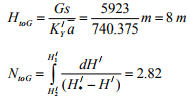Height of the cooling tower=8×2.82 m=22.56 m
(b) Overall gas-phase enthalpy transfer unit (NtoG)=2.82
(c) TL1 is unknown. Height of the cooling tower is same, i.e., 22.56 m. Slope of the operating line is as before, i.e., 6.006. TL1 should be greater than 29ºC. Assume TL1 as 32ºC.
Get height of the cooling tower. If height is 22.56 m, 32ºC is the answer. Otherwise guess another TL1.
Or,
TG1=32ºC, Tw1=25.5ºC, From psychrometric chart, Y/1 0.017 kg / kg dry air
H/1 = 75.682 KJ / kg
LcwL (TL2 - TL1) = Gs(H/2 - H/1)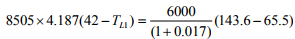TL1=30.8ºC

Offer running on EduRev: Apply code STAYHOME200 to get INR 200 off on our premium plan EduRev Infinity!

## Mass Transfer

29 videos|46 docs|44 tests

,

,

,

,

,

,

,

,

,

,

,

,

,

,

,

,

,

,

,

,

,

;